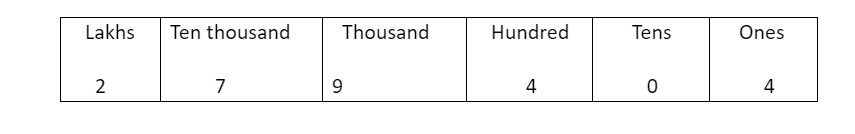QUESTION

# Write the following numbers in expanded form: 279404

Hint: Represent number in place value table and then try to answer the question, that is split the digits of the given numbers as per their place value.So, the digit 2 is in lakhs position, the digit 7 is in ten thousand, the digit 9 is in thousand, the digit 4 is in hundred, the 0 is in tens and finally 4 in ones place.
$279404=2\times 1,00,000+7\times 10,000+9\times 1,000+4\times 100+0\times 10+4\times 1$
Hence the answer is $279404=2\times 1,00,000+7\times 10,000+9\times 1,000+4\times 100+0\times 10+4\times 1$
$\Rightarrow 279404=2\times {{10}^{5}}+7\times {{10}^{4}}+9\times {{10}^{3}}+4\times {{10}^{2}}+0\times {{10}^{1}}+4\times {{10}^{\circ }}.$